xTras Tutorial | Functions »

# Symmetrized Covariant Derivatives

## Introduction

A symmetrized derivative covariant derivative is symmetrization of a number of covariant derivatives:The main advantage of symmetrized derivatives is that they have a greater degree of symmetry than non-symmetrized (or ordinary) derivatives. For instance, it is obvious that the following is zero:However, when we write out the symmetrized derivative, it is not immediately clear that the expression is zero: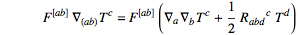Of course, when we writeas a commutator, we recover zero. But the point is that eliminating commutators is something the xTensor canonicalizer does not do. So it is often necessary to explicitly symmetrize covariant derivatives in order to fully canonicalize an expression.

## Implementation

 SymmetrizeCovDs symmetrizes covariant derivatives ExpandSymCovDs de-symmetrizes covariant derivatives \$AutoSymmetrizeCovDs when set to True, derivatives are automatically symmetrized

Functions for symmetrizing and de-symmetrizing covariant derivatives.

Symmetrized covariant derivatives are implement in xTras as follows. Whenever a covariant derivative CD is defined to be symmetrizable, CD[-a,-b] will mean(and likewise for more indices). A covariant derivative can be defined to symmetrizable by giving the option SymCovDQ -> True to DefCovD or DefMetric:
First define a manifold.
Now define a metric and covariant derivative. We need to specify SymCovDQ in order to make the covariant derivative symmetrizable.
Derivatives with more than one index are now interpreted as symmerized derivatives:
 Out=Out=We can expand the symmetrized derivative with a call to ExpandSymCovDs:
 Out=Single derivatives can be symmetrized with SymmetrizeCovDs:
 Out=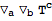Out=Symmetrized derivatives are automatically Leibnitz:
 Out=Integrating by parts can be done with VarD:
 Out=Notice that two symmetrized derivatives acting on each other are not automatically symmetrized further. We need to call SymmetrizeCovDs again to do so explicitly:
 Out=However, when we set \$AutoSymmetrizeCovDs to True, all derivatives that are not fully symmetrized will automatically get symmetrized:
 Out=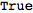Out=Out=Out=Because symmetrizing derivatives is a an operation the grows exponentially in complexity (see the section on performance below), the function SymmetrizeCovDs stores its results in the variable \$SymCovDCache. The cache can be cleared with ClearSymCovDCache:
 Out=By default, symmetrizations are cached:
 Out=Subsequent symmetrizations are then faster:
 Out=But the first symmetrization was slower compared to when no cache is used:
 Out=This is because SymmetrizeCovDs spents some time on store the results in the cache. If you plan on symmetrizing a lot of derivatives, using the cache is faster in the long run.

## Performance

The implementation of symmetrizing derivatives is exponentially complex in the number of derivatives. SymmetrizeCovDs has an optimized algorithm in the case when there is no torsion. Whenever there is torsion, a slower algorithm is used.## Properties of symmetrized derivatives

Below we list some useful formulas and properties of symmetrized derivatives.

### Symmetrizing

Because it is always possible to commute adjacent covariant derivatives by introducing curvature, a symmetrized covariant derivative can in general be expressed asor vice-versa:For example, we haveBy induction, we can deduce that a single ordinary derivative can be eaten by a symmetrized derivative as follows:where the general commutator operatoris given byAlthough it is not explicitly indicated after the last equal sign because it would clutter notation, all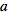indices above are symmetrized over.
This expression for the operatoris not completely closed; it contains terms of the formfor which it is quite hard to find a closed formula relating it to.
However, whenever there is no torsion, the operatorcan be expressed aswhere the commutator in the first term does not act on the indices of, but only on the expression on which the operatoracts. Furthermore, the numeric functionsare given bywhereis the UnitStep function. Note thatwith fixedandgets fed into the next iteration ofas its).
A similar closed form forwith torsion should also exist.

### Other properties

Distributivity is inherited from the ordinary derivative:If the ordinary derivative is Leibnitz, we have the following generalization of the Leibnitz rule:Counting the number of terms on boths sides provides a consistency check on this formula; the left-hand-side hasterms, the same as the right-hand-side, namely.
Integrating by parts also carries over nicely:Lastly, the action of a Leibnitz symmetrized derivative on a generic functionwith arguments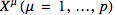can be written as the following generalized chain rule:This a generalization of Faà di Bruno's formula. Here, and the contracted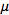indices are summed over. Furthermore,denotes the set of partitions ofintointegers(to be precise,is the result of IntegerPartitions[n, {i}]). Andis the weighted multinomialNote thatwhereis the Stirling number of the second kind, andis the Bell number.
The generalized Leibnitz rule above is actually a limiting case of the generalized chain rule, as we can see by setting:Similarly, the distributivity property can also be derived from the generalized chain rule by setting.